# Abstract Data Types

Abstract Data type (ADT) is a type (or class) for objects whose behaviour is defined by a set of value and a set of operations.

The definition of ADT only mentions what operations are to be performed but not how these operations will be implemented. It does not specify how data will be organized in memory and what algorithms will be used for implementing the operations. It is called “abstract” because it gives an implementation-independent view. The process of providing only the essentials and hiding the details is known as abstraction.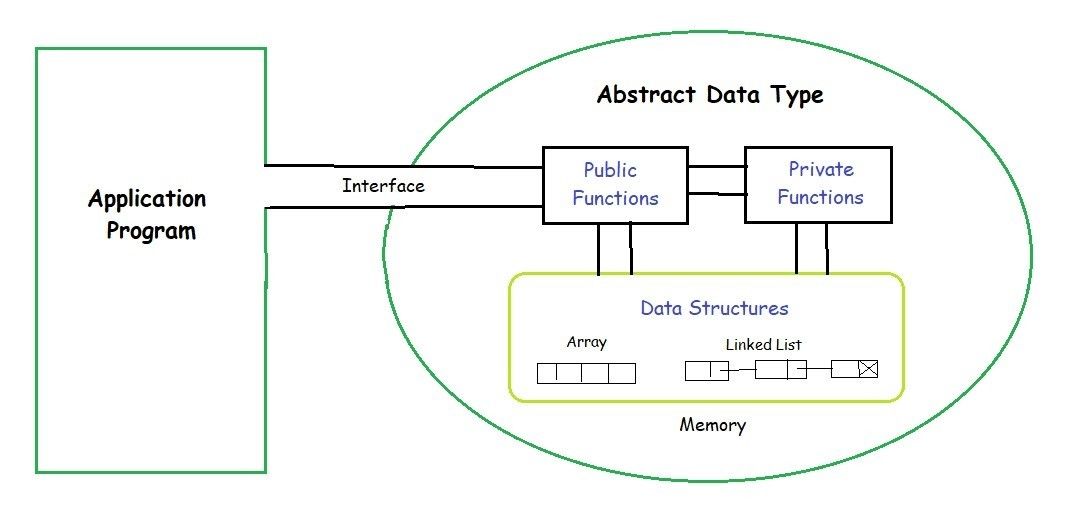The user of data type does not need to know how that data type is implemented, for example, we have been using Primitive values like int, float, char data types only with the knowledge that these data type can operate and be performed on without any idea of how they are implemented. So a user only needs to know what a data type can do, but not how it will be implemented. Think of ADT as a black box which hides the inner structure and design of the data type. Now we’ll define three ADTs namely List ADT, Stack ADT, Queue ADT.

• The data is generally stored in key sequence in a list which has a head structure consisting of count, pointers and address of compare function needed to compare the data in the list.
•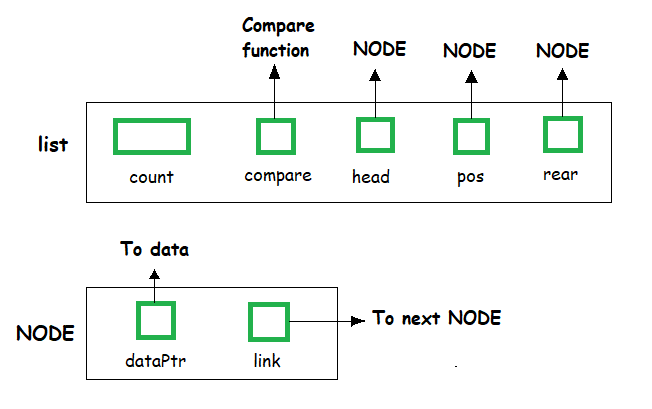• The data node contains the pointer to a data structure and a self-referential pointer which points to the next node in the list.
•  `//List ADT Type Definitions ` `typedef` `struct` `node ` `{ ` ` ``void` `*DataPtr; ` ` ``struct` `node *link; ` `} Node; ` `typedef` `struct` `{ ` ` ``int` `count; ` ` ``Node *pos; ` ` ``Node *head; ` ` ``Node *rear; ` ` ``int` `(*compare) (``void` `*argument1, ``void` `*argument2) ` `} LIST;  `

• The List ADT Functions is given below:
•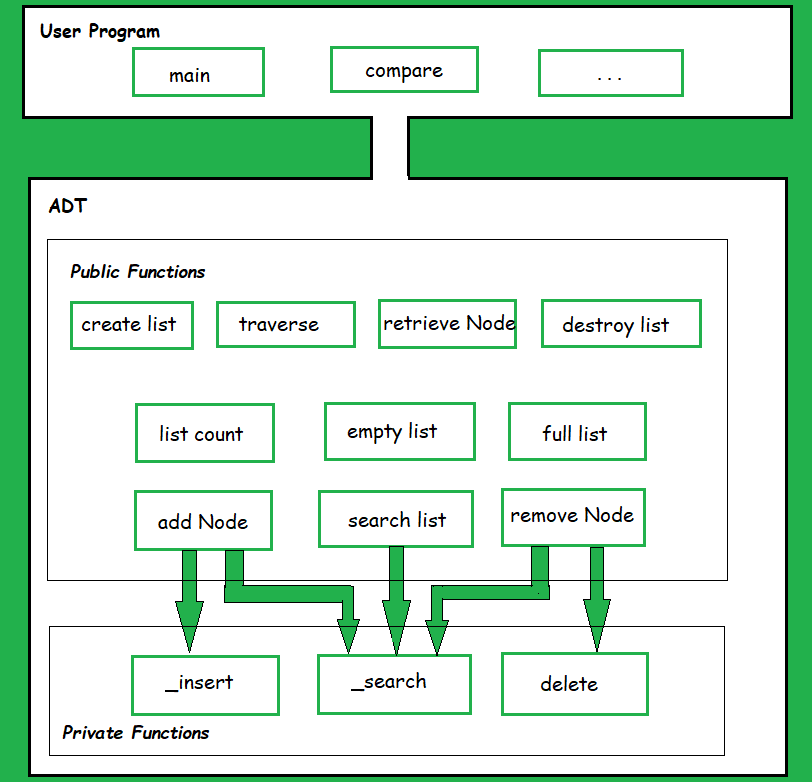A list contains elements of the same type arranged in sequential order and following operations can be performed on the list.

• get() – Return an element from the list at any given position.
• insert() – Insert an element at any position of the list.
• remove() – Remove the first occurrence of any element from a non-empty list.
• removeAt() – Remove the element at a specified location from a non-empty list.
• replace() – Replace an element at any position by another element.
• size() – Return the number of elements in the list.
• isEmpty() – Return true if the list is empty, otherwise return false.
• isFull() – Return true if the list is full, otherwise return false.

• In Stack ADT Implementation instead of data being stored in each node, the pointer to data is stored.
• The program allocates memory for the data and address is passed to the stack ADT.
•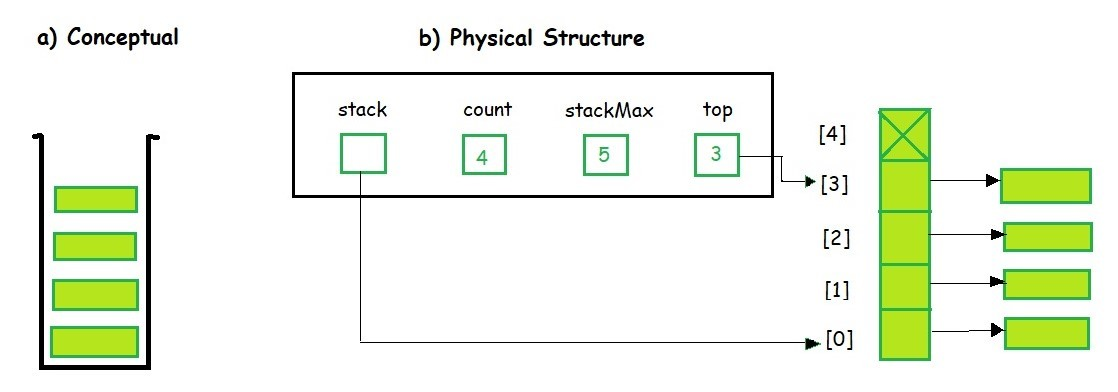• The head node and the data nodes are encapsulated in the ADT. The calling function can only see the pointer to the stack.
• The stack head structure also contains a pointer to top and count of number of entries currently in stack.
•  `//Stack ADT Type Definitions ` `typedef` `struct` `node ` `{ ` ` ``void` `*DataPtr; ` ` ``struct` `node *link; ` `} StackNode; ` `typedef` `struct` `{ ` ` ``int` `count; ` ` ``StackNode *top; ` `} STACK; `

A Stack contains elements of the same type arranged in sequential order. All operations take place at a single end that is top of the stack and following operations can be performed:

• push() – Insert an element at one end of the stack called top.
• pop() – Remove and return the element at the top of the stack, if it is not empty.
• peek() – Return the element at the top of the stack without removing it, if the stack is not empty.
• size() – Return the number of elements in the stack.
• isEmpty() – Return true if the stack is empty, otherwise return false.
• isFull() – Return true if the stack is full, otherwise return false.

• The queue abstract data type (ADT) follows the basic design of the stack abstract data type.
•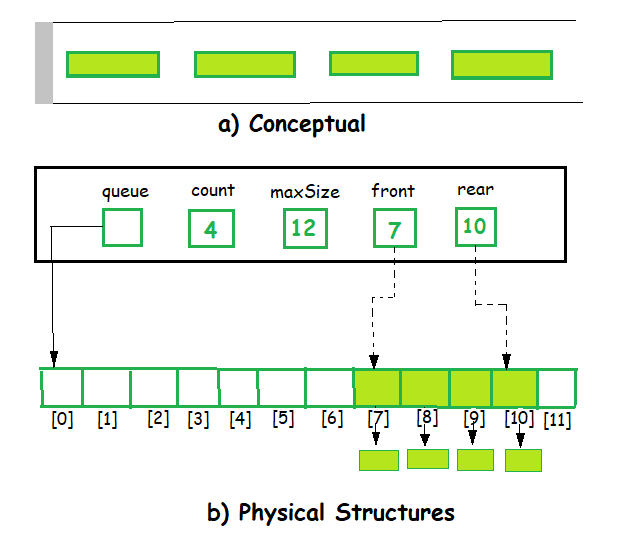• Each node contains a void pointer to the data and the link pointer to the next element in the queue. The program’s responsibility is to allocate memory for storing the data.
•  `//Queue ADT Type Definitions ` `typedef` `struct` `node ` `{ ` ` ``void` `*DataPtr; ` ` ``struct` `node *next; ` `} QueueNode; ` `typedef` `struct`  `{ ` ` ``QueueNode *front; ` ` ``QueueNode *rear; ` ` ``int` `count; ` `} QUEUE; `

A Queue contains elements of the same type arranged in sequential order. Operations take place at both ends, insertion is done at the end and deletion is done at the front. Following operations can be performed:

• enqueue() – Insert an element at the end of the queue.
• dequeue() – Remove and return the first element of the queue, if the queue is not empty.
• peek() – Return the element of the queue without removing it, if the queue is not empty.
• size() – Return the number of elements in the queue.
• isEmpty() – Return true if the queue is empty, otherwise return false.
• isFull() – Return true if the queue is full, otherwise return false.

From these definitions, we can clearly see that the definitions do not specify how these ADTs will be represented and how the operations will be carried out. There can be different ways to implement an ADT, for example, the List ADT can be implemented using arrays, or singly linked list or doubly linked list. Similarly, stack ADT and Queue ADT can be implemented using arrays or linked lists.

This article is contributed by Anuj Chauhan. If you like GeeksforGeeks and would like to contribute, you can also write an article using contribute.geeksforgeeks.org or mail your article to contribute@geeksforgeeks.org. See your article appearing on the GeeksforGeeks main page and help other Geeks.

Don’t stop now and take your learning to the next level. Learn all the important concepts of Data Structures and Algorithms with the help of the most trusted course: DSA Self Paced. Become industry ready at a student-friendly price.

My Personal Notes arrow_drop_up